### Home > MC2 > Chapter 9 > Lesson 9.2.3 > Problem9-64

9-64.
1. The perimeter of one shape is 5.5 times larger than the perimeter of a similar shape. Homework Help ✎

1. How many times larger are the sides of the large shape than the corresponding sides of the smaller shape?

2. How many times larger is the area?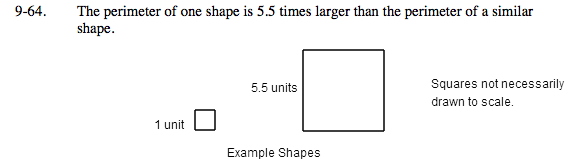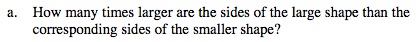According to the Math Notes box from Lesson 9.2.2: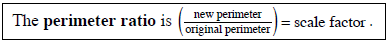Does this make sense? Find the perimeter of the two example shapes above and see if it follows this same rule.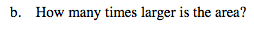According to the Math Notes box from Lesson 9.2.2: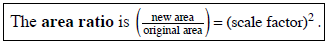Find the area of the two shapes and see if it follows this rule. What did you find?

30.25